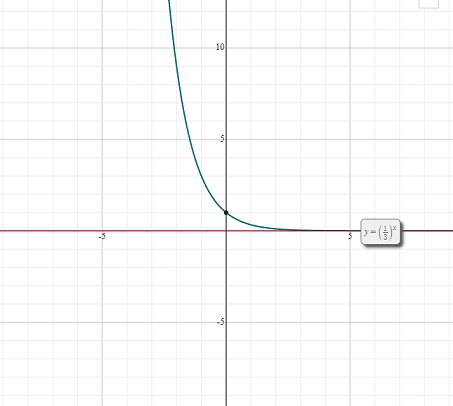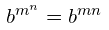## Exponential Functions

Exponential functions involve exponents also known as powers or indices. These functions are a little bit more complex than linear functions but much simpler than quadratic functions which are second degree polynomials. You might be thinking that every function that involves an exponent is an exponential function, however, it is not always the case. Consider the function. It involves an exponent 2, but it is not an exponential function, rather it is a parent quadratic function.

So, you might be wondering what exponential functions are and how they differ from any function which involves exponent? Well, the answer is quite simple. Remember the following definition of exponential functions and you will never be confused again.

Exponential functions are the functions in which the independent variable is an exponent, i.e. it is raised to a power. Mathematical notation of exponential functions is given below:Here,is a dependent variable,is an independent variable andis the base. The parent exponential function is.

After linear functions, exponential functions are the most commonly used functions. Even their applications span to our daily life. The examples of daily application of exponential functions are given below:

• You will use exponential function if you want to represent the population growth in a city over a period of time
• Similarly, scientists use these functions to determine the exponential decay of radioactive elements
• Biologists can use exponential functions to compute the exponential growth of the population of algae in a targeted area

## Properties of Exponential Functions

In this section, we will discuss the properties of exponential functions with respect to the value of the base. Let us see what happens when the value ofin the functionis between 0 and 1.

• As the independent variableincreases, the dependent variableapproaches to 0
• As the independent variabledecreases, the dependent variablereaches to infinity
• Its graph is horizontally asymptotic along the- axis
• The function decreases strictly

Now, let us see an example of an exponential function whose base is between 0 and 1. Consider an exponential function. To graph this exponential function, we need to construct its table first.

xy
-29
-13
01
11/3
21/9

Graphing the above exponential function using the table of values, we get the following exponential curve:You can see that the above graph is satisfying all the properties of exponential functions having the base between 0 and 1 which were described above.

Now, we will see what are the properties of exponential functions having base greater than 1.

• As the independent variableincreases, the dependent variableapproaches to infinity
• As the independent variabledecreases, the dependent variableapproaches to 0
• This function strictly increases
• Its graph is also horizontally asymptotic along the- axis

You can see that the graphs of the functions having a base less than or greater than 1 both are asymptotic to the- axis.

Let us consider a function whose base is greater than 1. To graph this function, first, we need to construct a table of values for this function. The table with independent and dependent variables is given below:

xy
-21/9
-11/3
01
13
29

Using the above table of values, the graph of the functionis given below:You can see that the above graph satisfies all the properties which were listed for the exponential function having a base greater than 1.

## Exponent Rules

In this section, we will see what are the rules of exponents. These rules are very helpful in simplifying exponential functions that involve multiple operations of addition, subtraction, multiplication or division.

### Exponent Product Rule

When two exponential expressions with a common base are multiplied with each other, they can be written as a single exponential function with their exponents added together while keeping the same base. Mathematically it is represented as follows:### Example

Evaluate.

As both the exponential functions have same base 3, so the resultant expression will be:### Exponent Quotient Rule

Exponent quotient rule says that when two exponential functions with the same base are divided by each other, they can be written as a single exponential function with the same base but the power equal to the difference between the exponents. Mathematically it is denoted as:### Example

EvaluateAs both the exponential functions have the common base 5, the resultant expression will be:### Exponent Power Rule

According to the exponent power rule, if the exponential function has two powers, i.e. the power of the expression also has a power, then we first multiply the powers and then the base is solved for the resultant power. It can be written mathematically as:### Example

SolveApplying the exponential power rule, we will write the expression as:We know thatis equal to 4, so the expression will be:### Exponential Power of Product Rule

According to the exponential power of product rule, the product of two numbers with a common power can be written as two separate exponential functions. It can be expressed mathematically as:### Example

EvaluateAccording to the exponent power of product rule, this expression can be written as:### Exponent Power of a Fraction Rule

According to a power of a fraction rule, the division of two numbers with a common power can be written as the division of two separate exponential functions. It can be expressed mathematically as:### Example

Evaluate.

According to the exponent power of fraction rule, this expression can be written as:### Zero Exponent Rule

According to zero exponent rule, a number having the power zero is equal to 1. It can be expressed mathematically as:### Example

EvaluateAccording to the zero exponent rule,### Negative Exponent Rule

According to negative exponent rule, a number with a negative power is equal to the reciprocal of the number with positive power. It means that if there is a negative exponent in the denominator, then it will move to the numerator and negative exponent will change to a positive one. Similarly, if there is a negative exponent in the numerator, then it will move to the denominator and the exponent will become positive. It can be expressed mathematically as:### Example

EvaluateAccording to negative exponent rule,can be written as:### Fractional Exponent Rule

According to the fractional exponent rule, a number having a fraction as an exponent can be expressed as a number with root. It can be expressed mathematically as:### Example

EvaluateAccording to the fractional exponent rule,can be written as:### Exponent Rule Summary Table

The summary table of all the above laws is given below:

Exponent RulesMathematical Notation
Product ruleQuotient rulePower rulePower of product rulePower of fraction ruleZero exponent ruleNegative exponent ruleFractional exponent rule## Steps in Simplifying the Exponential Function

Now that we are familiar with the exponent rules, we will discuss the steps that should be followed to simplify a complex exponential function. Remember that you need to use the exponent rules in order to solve the complex exponential functions.

### Step 1

First, you need to apply a zero - exponent rule which says that anything having a zero power is equal to 1.

### Step 2

After applying the zero - exponent rule, use power rule. According to the power rule, if a number contains two powers, i.e. the exponent further has another exponent, then we multiply both the powers while keeping the base same.

### Step 3

The next step is to apply a negative exponent rule. According to this rule, a negative exponent in the numerator becomes the positive exponent after moving to the denominator and vice versa. Remember that you cannot move positive exponents. As the name implies this rule is applicable to negative exponents only.

### Step 4

Apply product rule which says that if the exponents with the same base are multiplied with each other, their exponents are added together while the base remains the same.

### Step 5

Apply the quotient rule which says that when two exponents with the same base are divided by each other, we keep the base and take the difference of the exponents. Remember that the exponent product and quotient rules do not apply to the expressions with different bases.

### Step 6

Simplify the remaining fractions by writing an appropriate power of the coefficients.

## Simplifying Complex Exponential Function

The following examples indicate how to simplify the exponential functions using sequential exponent rules.

### Example 1

Simplify the expression### Solution

To simplify the above expression, follow the exponent rules in order. The first step was to apply zero exponent rule. Since, no number is raised to the power 0, so we will directly move to the next step which directs to apply power rule. After applying the power rule, the resulting expression will be as follows:The next step is to apply negative exponent rule. You can see that all the factors have negative powers with them, so we will take the reciprocal of the whole expression. The resultant expression will be:Now, apply the product rule. Since, there are no exponents with same base in numerator or denominator, so we will move to the quotient rule.### Example 2

Simplify the expression### Solution

Since, there is no number or variable in the above expression, which is raised to the power 0, so we will directly move to the next step which is applying the power rule. The resultant expression after applying the power rule will be:Now, the next step is to apply the negative exponent rule. According to the negative exponent rule, any number or variable with a negative power in the numerator will go to the denominator and become positive and vice versa.Now, the next step is to apply product rule. Since, there are no common expressions in the numerator or denominator, so we will skip this step and the next step which directed to apply the quotient rule. Simply, simplify the expression to get the final answer:### Example 3

Simplify the expression### Solution

Currently, there is no variable in the expression which is raised to the power zero, so we will move to the next step which directs to apply power rule. The expression after applying the power rule is given below::Now, the next step is to apply the negative exponent rule. According to this rule, if there is any base with a negative exponent in the numerator, we will write it in the denominator and change the sign of exponent from positive to negative and vice versa.Now, we will see if we can apply the product rule which says that the bases with same exponents can be written as a one base with its exponent equal to the addition of two bases. Since, there are no bases to which we can apply the product rule, so we will move to the next step.

The next step directs us to apply the quotient rule which says that exponents with the same bases in the numerator and denominator can be written as the the same base with its exponent equal to the difference of exponents.Here, we need to apply the zero exponent rule because we have gotraised to the power zero after simplifying.Hence, the simplified form of the expression will be:Did you like the article?(1 votes, average: 5.00 out of 5)Loading...

Emma

I am passionate about travelling and currently live and work in Paris. I like to spend my time reading, gardening, running, learning languages and exploring new places.

Did you like
this resource?

Bravo!